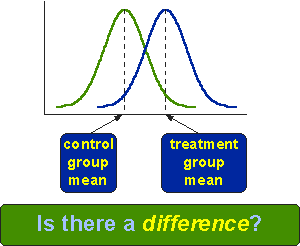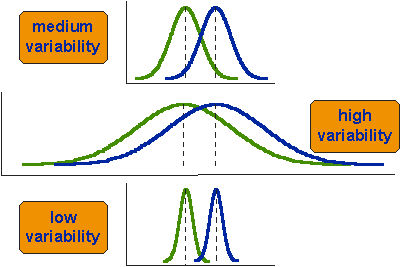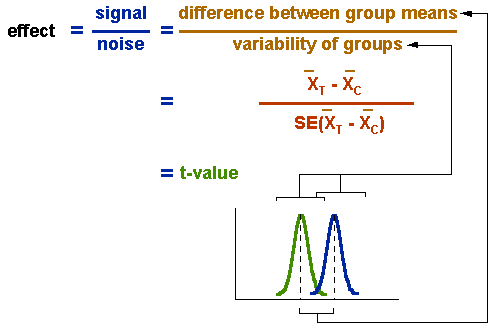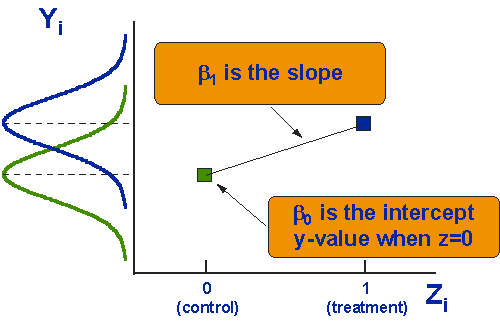Posttest-Only Analysis

# Posttest-Only Analysis

To analyze the two-group posttest-only randomized experimental design we need an analysis that meets the following requirements:

• has two groups
• uses a post-only measure
• has two distributions (measures), each with an average and variation
• assess treatment effect = statistical (i.e., non-chance) difference between the groupsBefore we can proceed to the analysis itself, it is useful to understand what is meant by the term “difference” as in “Is there a difference between the groups?” Each group can be represented by a “bell-shaped” curve that describes the group’s distribution on a single variable. You can think of the bell curve as a smoothed histogram or bar graph describing the frequency of each possible measurement response. In the figure, we show distributions for both the treatment and control group. The mean values for each group are indicated with dashed lines. The difference between the means is simply the horizontal difference between where the control and treatment group means hit the horizontal axis.Now, let’s look at three different possible outcomes, labeled medium, high and low variability. Notice that the differences between the means in all three situations is exactly the same. The only thing that differs between these is the variability or “spread” of the scores around the means. In which of the three cases would it be easiest to conclude that the means of the two groups are different? If you answered the low variability case, you are correct! Why is it easiest to conclude that the groups differ in that case? Because that is the situation with the least amount of overlap between the bell-shaped curves for the two groups. If you look at the high variability case, you should see that there quite a few control group cases that score in the range of the treatment group and vice versa. Why is this so important? Because, if you want to see if two groups are “different” it’s not good enough just to subtract one mean from the other – you have to take into account the variability around the means! A small difference between means will be hard to detect if there is lots of variability or noise. A large difference will between means will be easily detectable if variability is low. This way of looking at differences between groups is directly related to the signal-to-noise metaphor – differences are more apparent when the signal is high and the noise is low.With that in mind, we can now examine how we estimate the differences between groups, often called the “effect” size. The top part of the ratio is the actual difference between means, The bottom part is an estimate of the variability around the means. In this context, we would calculate what is known as the standard error of the difference between the means. This standard error incorporates information about the standard deviation (variability) that is in each of the two groups. The ratio that we compute is called a t-value and describes the difference between the groups relative to the variability of the scores in the groups.

There are actually three different ways to estimate the treatment effect for the posttest-only randomized experiment. All three yield mathematically equivalent results, a fancy way of saying that they give you the exact same answer. So why are there three different ones? In large part, these three approaches evolved independently and, only after that, was it clear that they are essentially three ways to do the same thing. So, what are the three ways? First, we can compute an independent t-test as described above. Second, we could compute a one-way Analysis of Variance (ANOVA) between two independent groups. Finally, we can use regression analysis to regress the posttest values onto a dummy-coded treatment variable. Of these three, the regression analysis approach is the most general. In fact, you’ll find that I describe the statistical models for all the experimental and quasi-experimental designs in regression model terms. You just need to be aware that the results from all three methods are identical.

$$y_{i}=\beta_{0}+\beta_{1} Z_{i}+e_{i}$$

where:

• yi is the outcome of the ith unit
• β0 = coefficient for the intercept
• β1 = coefficient for the slope
• zi = 1 if ith unit is in the treatment group,
= 0 if ith unit is in the control group
• ei = residual for the ith unit

OK, so here’s the statistical model in notational form. You may not realize it, but essentially this formula is just the equation for a straight line with a random error term thrown in ei. Remember high school algebra? Remember high school? OK, for those of you with faulty memories, you may recall that the equation for a straight line is often given as:

$$y = mx + b$$

which, when rearranged can be written as:

$$y = b + mx$$

(The complexities of the commutative property make you nervous? If this gets too tricky you may need to stop for a break. Have something to eat, make some coffee, or take the poor dog out for a walk.). Now, you should see that in the statistical model yi is the same as y in the straight line formula, b0 is the same as b, b1 is the same as m, and Zi is the same as x. In other words, in the statistical formula, b0 is the intercept and b1 is the slope.It is critical that you understand that the slope, b1 is the same thing as the posttest difference between the means for the two groups. How can a slope be a difference between means? To see this, you have to take a look at a graph of what’s going on. In the graph, we show the posttest on the vertical axis. This is exactly the same as the two bell-shaped curves shown in the graphs above except that here they’re turned on their side. On the horizontal axis we plot the Z variable. This variable only has two values, a 0 if the person is in the control group or a 1 if the person is in the program group. We call this kind of variable a “dummy” variable because it is a “stand in” variable that represents the program or treatment conditions with its two values (note that the term “dummy” is not meant to be a slur against anyone, especially the people participating in your study). The two points in the graph indicate the average posttest value for the control (Z=0) and treated (Z=1) cases. The line that connects the two dots is only included for visual enhancement purposes – since there are no Z values between 0 and 1 there can be no values plotted where the line is. Nevertheless, we can meaningfully speak about the slope of this line, the line that would connect the posttest means for the two values of Z. Do you remember the definition of slope? (Here we go again, back to high school!). The slope is the change in y over the change in x (or, in this case, Z). But we know that the “change in Z” between the groups is always equal to 1 (i.e., 1 - 0 = 1). So, the slope of the line must be equal to the difference between the average y-values for the two groups. That’s what I set out to show (reread the first sentence of this paragraph). b1 is the same value that you would get if you just subtract the two means from each other (in this case, because we set the treatment group equal to 1, this means we are subtracting the control group out of the treatment group value. A positive value implies that the treatment group mean is higher than the control, a negative means it’s lower). But remember at the very beginning of this discussion I pointed out that just knowing the difference between the means was not good enough for estimating the treatment effect because it doesn’t take into account the variability or spread of the scores. So how do we do that here? Every regression analysis program will give, in addition to the beta values, a report on whether each beta value is statistically significant. They report a t-value that tests whether the beta value differs from zero. It turns out that the t-value for the b1 coefficient is the exact same number that you would get if you did a t-test for independent groups. And, it’s the same as the square root of the F value in the two group one-way ANOVA (because t2 = F).

Here’s a few conclusions from all this:

• the t-test, one-way ANOVA and regression analysis all yield same results in this case
• the regression analysis method utilizes a dummy variable (Z) for treatment
• regression analysis is the most general model of the three.